# 10 Programming questions and exercises for Java Programmers

If you have just started learning basics of Java programming language or familiar with programming in either C or C++ then these Java programming questions and exercises for you. It doesn't focus on particular part of Java but these coding exercises will switch you in programming mode.These are also great way to master basic programming construct like if-else , loops like for and while,Java operators e.g. arithmetic and logical operator, recursion, methods or functions and common Java API. You may also find these Java programming questions in most of Java courses taught in school, colleges and various Java training courses. Even I have started learning Java by doing these exercises multiple times with different ways. They are interesting, gives feeling of accomplishment if you complete it. These Java programs looks simple but they are still tricky for novice Java programmers. Try to solve these coding exercises by yourself but if you stuck you can check relevant links or of-course use google to get more insight on them. You can also see here more Java programming questions and exercises.

## Java Programing Questions exercises for beginners to practices

Here is my list of 10 Java programming questions or Java programs which can help any beginner to get started in programming world. These are classics, popular and very effective. You can use either notepad or any Java IDE like Eclipse or Netbeans for coding. See links for solution and hints.

1. Write a program in Java to check if a number is even or odd in Java? (input 2 output true, input 3 : output false)
A number is called even if it is completely divisible by two and odd if it’s not completely divisible by two. For example number 4 is even number because when you do 4/2 , remainder is 0 which means 4 is completely divisible by 2. On the other hand 5 is odd number because 5/2 will result in remainder as 1. See here

2. Write a program in Java to find out if a number is prime in Java? (input 7: output true , input 9 : output false)
A number is called prime if it is divisible by either itself or 1. There are many algorithm to find prime numbers e.g. instead of dividing till number, division upto square root of number may be enough. Start from simplest one and than try to solve this problem with couple of different ways. Here is one way to check prime numbers in Java

3. Write Java program to check if a number is palindrome in Java? ( 121 is palindrome, 321 is not)
A number is called a palindrome if number is equal to reverse of number e.g. 121 is palindrome because reverse of 121 is 121 itself. On the other hand 321 is not palindrome because reverse of 321 is 123 which is not equal to 321. See here for solution

4. How to find if a number is power of 2 in Java? (1,2, 4 power of 2, 3 is not)
This is another interesting Java programming exercise. This program can be solved using different ways e.g. using arithmetic operator or by using bit shift operator.

5. Write program to sort an integer array without using API methods?Sorting questions are one of the integral part of programming questions. There are many sorting algorithm out there to sort any array in Java e.g. Bubble sort, Insertion sort, Selection sort or quick sort. Implementing sorting algorithm itself a good programming exercise in Java. By the way here is one way to sort an integer array with Bubble sort algorithm in Java.

6. Write Java program to check if a number is Armstrong number or not? (input 153 output true,  123 output false)
An Armstrong number of 3 digit is a number for which sum of cube of its digits are equal to number e.g. 371 is an Armstrong number because 3*3*3 + 7*7*7 + 1*1*1 = 371). See here for sample Java pro

7. Write a program in Java to reverse any String without using StringBuffer?
This is another classical Java programming question. You can reverse String in various way in Java but two programming technique is used to do e.g. Iteration and Recursion. Try solving this problem using Iteration first by using Java’s arithmetic operator and than look to implement a recursive solution. Here is one way to reverse String in Java without using StringBuffer.

8. Write a program in Java to print Fibonacci series up to given number? Write both iterative and recursive version.
Fibonacci series a popular number series and very popular programming question in Java, in which number is equal to sum of previous two numbers, starting from third. Fibonacci series is also a good recursion exercise and often asked in Interviews as well. Try doing this exercise by using both Iteration e.g. loops and recursion. For

9. Write a Java program to calculate factorial of an integer number ? Both iterative and recursive solution.
Calculating Factorial is also a classic recursion exercise in programming. Since factorial is a recursive function, recursion becomes natural choice to solve this problem. You just need to remember formula for calculating factorial which is for n! its n*(n-1)*…1. here is one wa

10. Print following structure in Java?
This program is a good exercise for mastering loops e.g. for loop and while loop in Java. This also teaches y By the way you can print any character and use System.out.print() and System.out.println())
*
***
*****
***
*

These were some programming questions and exercises for beginners learning Java programming language. This list is simple and you can solve these coding exercises in any programming language. I am sure Java beginners will find these exercises interesting and useful. You can also post any coding exercise which you think can help junior programmers to learn programming and help converting logic to code.

Further Learning
The Coding Interview Bootcamp: Algorithms + Data Structures
Data Structures and Algorithms: Deep Dive Using Java
Algorithms and Data Structures - Part 1 and 2

Other Java programming tutorials for beginners

#### 51 comments:

1.These are good Java programming exercises for beginners but for experienced programmer its too simple. I would rather give complex application to develop to experienced programmer in couple of hours rather than simple programming exercise.

1.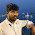can you send me please some complex program exercise on my email:arun.rout31@gmail.com plz plzzz

2.yes why not dude. please contact me via mustafaaftab2624@gmail.com

2.To the previous commenter, even for experienced programmers some of these problems are still usefull to sharpen your skills with. A solid base will improve your overall ability and infact research into finding an efficint method of finding the factorial of an integer is still being conducted, if I'm not mistaken.

3.A good one would be to create an alarm clock that can work in both letters and numbers. Another would be fixing code for a program you garbled. Still, these are good school style exercises! Thank you.

4.I was looking for some beginners Java questions for my training course. I loved your collection, they are not too difficult, easy to understand but only thing is that they lack novelty. They are quite old Java questions. Though I would like to use these, I am also putting my own e.g.

1) Write a Java program to get all hashtags and mentioned in a twitter message? (This would be a good String matching exercise in Java, as you can use regular expression, can split string etc. As hashtags starts with '#' and mentioned strats with '@' character.

2) Count How many characters a String contains, without including any white space e.g. \t \n etc.

1.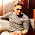can you show the output of 1st programme

5.Admin can you post more Java programs which are used for improving programming skill and also useful for interviews(these 10 questions are good but want more and more like such questions friend....)

6.Exercise 10 for anyone interested, simple version.

public class pyramidDrawing {

public static void main(String[] args) {

simpleDrawing(10);
arrayDrawing(10);

}

public static void simpleDrawing(int number) {
for(int i = 1; i <= number; i++) {
for(int j = 1; j <= i; j++) {
System.out.print("*");
}
System.out.println();
}
for(int v = number; v >= 1; v--) {
for(int q = 1; q <= v; q++) {
System.out.print("*");
}
System.out.println();
}
}

public static void arrayDrawing(int number) {
List numere = new ArrayList();

for(int numar = 1 ; numar <= number; numar++) {
numere.add(numar);
}

for(int i = 0; i < numere.size(); i++) {
if(i % 2 == 0) {
for(int j = 0; j <= i; j++) {
System.out.print(numere.get(i));
}
System.out.println();
}
}

for(int v = numere.size(); v >= 0; v--) {
if(v % 2 != 0) {
for(int q = 1; q <= v; q++) {
System.out.print(numere.get(v-1));
}
System.out.println();
}
}
}
}

1.*
**
***
****
*****
******
*******
********
*********
**********
**********
*********
********
*******
******
*****
****
***
**
*
1
333
55555
7777777
999999999
999999999
7777777
55555
333
1

2.Updated one, as we no need to repeat the biggest number of stars or number for example 999999999, and also the argument in simpleDrawing(10) has to be 1,3,5,7,9,11or so on not 10
import java.util.ArrayList;
import java.util.List;

public class pyramidDrawing {

public static void main(String[] args) {

simpleDrawing(7);
arrayDrawing(10);

}

public static void simpleDrawing(int number) {
for(int i = 1; i <= number; i+=2) {
for(int j = 1; j <= i; j++) {
System.out.print("*");
}
System.out.println();
}
for(int v = number-2; v >= 1; v-=2) {
for(int q = 1; q <= v; q++) {
System.out.print("*");
}
System.out.println();
}
}

public static void arrayDrawing(int number) {
List numere = new ArrayList();

for(int numar = 1 ; numar <= number; numar++) {
numere.add(numar);
}

for(int i = 0; i < numere.size(); i++) {
if(i % 2 == 0) {
for(int j = 0; j <= i; j++) {
System.out.print(numere.get(i));
}
System.out.println();
}
}

for(int v = numere.size()-2; v >= 0; v--) {
if(v % 2 != 0) {
for(int q = 1; q <= v; q++) {
System.out.print(numere.get(v-1));
}
System.out.println();
}
}
}
}

7.Dear admin,please give me the answer of no6..

1.import java.util.Scanner;

public class ArmstrongNumber {

public static void main(String[] args)
{
int org, temp , sum = 0 , r;

Scanner in = new Scanner(System.in);
System.out.println("Enter the number to find its armstrong number or not");
org = in.nextInt();

// System.out.println(org);
temp = org;

while (temp != 0)
{
r =temp % 10;

sum = sum +(r*r*r);

temp = temp - r;

temp = temp / 10;
}

if (org == sum)
{
System.out.println("the number " + org+ " is armstrong number");

}

else
{
System.out.println("the number " + org+ " is not armstrong number");

}

}

}

2.public class ArmstrongNo {
public static void main(String args[]) {
double n,a,b,c,d,e,f;
double g,h;
System.out.print("enter n");
n=TextIO.getInt();
g=n/100;
a=Math.floor(g);
d=n-100*a;
h=d/10;
b=Math.floor(h);
e=d-10*b;
c=e;
f=Math.pow(a,3)+Math.pow(b,3)+Math.pow(c,3);
if (f==n){
System.out.println("Yes");
}
else {
System.out.println("No");
}
}}

8.My solution for part 4, im a beginner.

import java.util.Scanner;
public class Tutorial {

public static void main(String[] args) {
Scanner sc = new Scanner(System.in);
System.out.println("compare if Power of 2");
while(true){
System.out.println("Input your number:");
double num = sc.nextInt();

double power=1;
int j=0;
for(int i=0;i<100;i++){
power=Math.pow(2, i);
//System.out.println("num = "+power);
if (num==power){
j++;
}

}
if (j>0){
System.out.println("IS a power of 2");
}
else
System.out.println("NOT a power of 2");

}
}
}

1.if you try to keep dividing the number by 2 and the number perfectly divided by 2 with 0 as remainder until you get 1 in the end then it is power of 2 otherwise it is not.

import java.util.Scanner;

public class PowerOfTwo {

public static void main(String[] args) {
// TODO Auto-generated method stub
Scanner scan = new Scanner(System.in);
int num = scan.nextInt();
while(num%2==0 && num!=0)
{
num = num/2;
}
if(num == 1)
System.out.println("Number is power of 2");
else
System.out.println("Number is not power of 2");
}

}

9.Too easy programs...........

10.A Dynamic Solution:
public class PrintStructure {

public static final int START = 1;
public static final int MAX = 10;
public static final int STEP_SIZE = 3;

public static void main(String[] args) {

int noOfLoops = (MAX - START) / STEP_SIZE;
noOfLoops++;
int noOfPrintingStars = START;
boolean startDecreasing = false;
for (int i = 0; i < (noOfLoops * 2 - 1); i++) {

for (int j = 0; j < noOfPrintingStars; j++) {

System.out.print("*");
}
System.out.println();

if (startDecreasing) {
noOfPrintingStars = noOfPrintingStars - STEP_SIZE;

} else {
noOfPrintingStars = noOfPrintingStars + STEP_SIZE;
}
if (noOfPrintingStars == MAX) {
startDecreasing = true;
}
}
}
}

11.3. Write a program that reads from the user four integers representing the numerators and denominators of two fractions, calculates the results of the two fractions and displays the values of the fractions sum, subtraction, multiplication and division.

12.3. Write a program that reads from the user four integers representing the numerators and denominators of two fractions, calculates the results of the two fractions and displays the values of the fractions sum, subtraction, multiplication and division.

Sample run:
Enter the numerator and denominator of the first fraction: 6 4
Enter the numerator and denominator of the second fraction: 8 5
The sum is: 3.1
The subtraction is: -0.1
The multiplication is: 2.4
The division is: 0.9375

13.2. Write a program that reads in from the user an integer (num) between 1000 and 9999. Then it prompts the user to enter an integer (d) between 0 and 9 and a character (ch). Your program should replace the second and the last digit in num with d and it should display the character that precedes (ch) followed by the number after the change and then the character that comes after (ch). Use the division and modulus operators to extract the digits from num.

Sample run:
Enter an integer between 1000 and 9999: 2134
Enter a digit (between 0 and 9): 6
Enter a character: b
Number was 2134. Result: a2636c.

1.import java.io.*;
import java.text.*;

public class Fraction
{
public static void main(String a[])throws IOException
{
DataInputStream r = new DataInputStream(System.in);

System.out.print("Enter the numerator and denominator of the first fraction : ");
String firstNum = r.readLine();

System.out.print("Enter the numerator and denominator of the second fraction : ");
String secondNum = r.readLine();

ComputeFraction cf = new ComputeFraction(firstNum,secondNum);

System.out.println("The sum is: " + cf.getSum());
System.out.println("The subtraction is: " + cf.getDiff());
System.out.println("The multiplication is: " + cf.getProduct());
System.out.println("The division is: " + cf.getQuotient());
}
}

class ComputeFraction
{
DecimalFormat df = new DecimalFormat("#.##");
double num1;
double num2;

public ComputeFraction(String firstNum, String secondNum)
{
String arr1[] = firstNum.split(" ");
String arr2[] = secondNum.split(" ");

num1 = Double.parseDouble(arr1) / Double.parseDouble(arr1);
num2 = Double.parseDouble(arr2) / Double.parseDouble(arr2);
}

public double getSum()
{
return Double.parseDouble(df.format(num1+num2));
}

public double getDiff()
{
return Double.parseDouble(df.format(num1-num2));
}

public double getProduct()
{
return Double.parseDouble(df.format(num1*num2));
}

public double getQuotient()
{
return Double.parseDouble(df.format(num1/num2));
}
}

2.Solution for Question 2:

import java.util.ArrayList;
import java.util.Scanner;

class Actions{

@SuppressWarnings("unused")
private int num;
@SuppressWarnings("unused")
private int d;
@SuppressWarnings("unused")
private char ch;

public void perform(int num, int d, char ch) {

this.num=num;
this.d=d;
this.ch=ch;

ArrayList list =new ArrayList();
String [] numberString = Integer.toString(num).split("");

list.add(Character.toString((char) (ch-1)));
list.add(numberString);
list.add(Integer.toString(d));
list.add(numberString);
list.add(Integer.toString(d));
list.add(Character.toString((char) (ch+1)));
StringBuilder result= new StringBuilder();
for (String s: list)
result.append(s);

System.out.println("Temp Result : "+result);
}

}

public class Tricky {

public static void main (String[] args ){

System.out.println("Please enter Number between 1000 to 9999 :");
@SuppressWarnings("resource")
int num = new Scanner(System.in).nextInt();
System.out.println("Please enter Number between 0 to 9 :");
@SuppressWarnings("resource")
int d = new Scanner(System.in).nextInt();
System.out.println("Please enter character :");
@SuppressWarnings("resource")
char ch = new Scanner(System.in).next().charAt(0);

@SuppressWarnings("rawtypes")
Actions acs = new Actions();
acs.perform(num,d,ch);

}
}

14.1. Write a program that takes three double values x0, v0, and t from the user and prints the value x0 +v0t +g t2/2, where g is the constant 9.78033. This value is the displacement in meters after t seconds when an object is thrown straight up from initial position x0 at velocity v0 meters per second.

Sample run:
Enter the value of x0, v0 and t: 0 2 2
The displacement in meters after 2 seconds when an object is thrown straight up from initial position 0 at velocity 2meters per second is: 23.56066

15.public static void printPyramid(int n) {
char s = '*';
for (int i = 1; i <= n/2; i++) {
for(int j=(i*2-1);j>0;j--){
System.out.print("* ");
}
System.out.println();
}
for (int i = n/2+1; i >= 0; i--) {
for(int j=(i*2-1);j>0;j--){
System.out.print("* ");
}
System.out.println();
}
}

16.Whats the answer for the second one?

17.Can you post string related coding question? Please send to this mail: vanitha23govindhan@gmail.com

18.here is my list of programs for practice
list of Java Program

Java Program to convert celsius to Farenheit and vice-versa
area of circle
perimeter of circle
are of rectangle
perimeter of rectangle
print alphabets
print multiplication table
largetst of three integers
floyd's triangle
pascal triangle
add matrices
transpose matrix
multiply matrix
perfect number or not
find common elements between two arrays
binary to decimal conversion
inverse of matrix

19.exercise for anyone interested?
1. Write a program that will print the following output

1
1 2 1
1 2 4 2 1
1 2 4 8 4 2 1
1 2 4 8 16 8 4 2 1
1 2 4 8 16 32 18 8 4 2 1

exercise number 2

2. Write a class named “Stock” to model a stock. The properties and methods of the class are shown in figure below. The method “changePercent” computes the percentage of the change of the current price vs the previous closing price. Write a client program to test the “Stock” class. In the client program, create a Stock object with the stock symbol SUNW, name Sun Microsystem Inc, previous closing price of 100. Set a new current price randomly and display the price change percentage.

Stock

private String symbol
private String name
private double previousClosingPrice

private double currentPrice
public Stock()
public Stock(String symbol, String name)

public String getSymbol()
public String getName()
public double getPreviousClosingPrice()
public double getCurrentPrice()
public void setSymbol(String symbol)
public void setName(String name)
public void setPreviousClosingPrice(double price)
public void setCurrentPrice(double price)
public double changePercent()

20.1. Write a program that will print the following output
1
1 2 1
1 2 4 2 1
1 2 4 8 4 2 1
1 2 4 8 16 8 4 2 1
1 2 4 8 16 32 18 8 4 2 1

1.import java.util.Scanner;

public class PracticeDemo {

public static void getNumber(int number){
int middle=1;
String start="1",end="1",output;
System.out.println(start);
for(int i=1;i<=number;i++){
middle=middle*2;
output=start+" "+middle+" "+end;
System.out.println(output);
start=start+" "+middle;
end=middle+" "+end;
}

}

public static void main(String [] args){
Scanner sc=new Scanner(System.in);
System.out.println("Enter the number");
int x=sc.nextInt();
getNumber(x);
sc.close();
}

}

output:
1
1 2 1
1 2 4 2 1
1 2 4 8 4 2 1
1 2 4 8 16 8 4 2 1
1 2 4 8 16 32 16 8 4 2 1
1 2 4 8 16 32 64 32 16 8 4 2 1
1 2 4 8 16 32 64 128 64 32 16 8 4 2 1
1 2 4 8 16 32 64 128 256 128 64 32 16 8 4 2 1
1 2 4 8 16 32 64 128 256 512 256 128 64 32 16 8 4 2 1
1 2 4 8 16 32 64 128 256 512 1024 512 256 128 64 32 16 8 4 2 1

2.I would say we should have i<number, instead of i<=number , So that the correct number of rows will print

3.yes you are right.

21.Program#1
//for beginners
Question: Write a program to count and print the frequency of word in a sentence(both accepted from the user)
For example:Inputs//sentence-The lazy lion jumped over the the sharp fence) just kidding!
word to be searched-the(irrespective of the case)
The normal way of doing it wud be a little difficult nested loops and compare each letter after finding the match of the first letter. Ill show u my way of doing!!

import java.io.*;
class freqWORD
{
int count=0;
public void main()throws IOException
{
BufferedReader b=new BufferedReader (new InputStreamReader(System.in));
System.out.println("Enter a line");
String line=b.readLine();
System.out.println("Enter thew word to be searched");
String word=b.readLine();
line=" "+line +" " ;
line=line.replace(word, "0");
for(int i=0; i<line.length(); i++)
{
if(line.charAt(i)=='0'&&line.charAt(i+1)==' '&&line.charAt(i-1)==' ')
{
count++;
}
}
System.out.println("the word is repeated " +count +" times");
System.out.println(line);

}
}

Better?!

1.The easiest way would be below:

import java.io.*;

class freqWORD
{

public static void main(String[] args)throws IOException
{
int count=0;
BufferedReader b=new BufferedReader (new InputStreamReader(System.in));
System.out.println("Enter a line");
String line=b.readLine();
System.out.println("Enter thew word to be searched");
String word=b.readLine();

String lines[] = line.split(" ");

for( String s: lines){

if (word.equals(s)){

count++;
}
}

System.out.println("the word is repeated " +count +" times");
System.out.println(line);

}
}

22.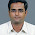Wap to find the product of first 20 numbers and display in proper format.

23.I have a ques. How to create application by taking integer to make palidrome coding....

24.Write a program in Java in DD array to convert all the elements into next prime numbers and display the changed array

1.Answer:

import java.io.BufferedReader;
import java.io.IOException;
import java.io.InputStreamReader;
import java.util.Arrays;

class Solution{

public int[] strArrayToIntArray(String[] a){
int[] b = new int[a.length];
for (int i = 0; i < a.length; i++) {
b[i] = Integer.parseInt(a[i]);
}

return b;
}

public int nextPrime(int num) {
num++;
for (int i = 2; i <num; i++) {
if(num%i == 0) {
num++;
i=2;
} else{
continue;
}
}
return num;
}

public int[] nextPrime(int[] data) {

for (int i=0;i<data.length;i++){

data[i]=nextPrime(data[i]);

}
return data;
}
}
public class NextPrime {

public static void main (String[] args) throws IOException{

System.out.println("Enter the numbers Separated space ");
BufferedReader br = new BufferedReader (new InputStreamReader(System.in));
String s =br.readLine();
String [] question = s.split(" ");
Solution sol = new Solution();
int[] data = sol.strArrayToIntArray(question);
int [] result = sol.nextPrime(data);
System.out.println("Result is :"+Arrays.toString(result));
}

}

25.write a program in java to accept a string from user by command line argument and display the vowel ?

1.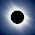Hello @Unknown, you can find the solution of displaying vowel or counting vowel here

2.Solution:

import java.io.BufferedReader;
import java.io.IOException;
import java.io.InputStreamReader;
import java.util.Arrays;

public class vowelCount {

public static void main (String [] args) throws IOException{

BufferedReader b=new BufferedReader (new InputStreamReader(System.in));
System.out.println("Enter a line");
String line=b.readLine();
String[] solution = line.split("(?!^)");
System.out.println("Word is :" +line);
System.out.println("Vowels are :");
for (String s :solution)
{
char c= s.charAt(0);
switch(c){

case 'a':
case 'e':
case 'i':
case 'o':
case 'u':
System.out.println(c);
break;
default:

}
}

}
}

26.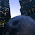public class Pattern {
public static void main(String args[]){
int[][] table = new int;

for(int row=0; row<table.length; row++) {
for(int col=0; col<table[row].length; col++){
System.out.print(" "+row*col+" ");

}
System.out.println();
}

}

}

output:
0 0 0 0
0 1 2 3
0 2 4 6
0 3 6 9

Output needed is:

0 1 2 3
1 1 2 3
2 2 4 6
3 3 6 9

what did i do wrong?

27.try below code...
for(int row=0; row<table.length; row++) {
System.out.print(row+" ");

for(int col=1; col<table[row].length; col++){
if(row!=0)
System.out.print(row*col+" ");
else
System.out.print(col+" ");

}
System.out.println();
}

1.Sir can you solve this problem? :

Write a java multiple choice examination program. with 10 questions only (need a specific question). Let the user choose answer and at the end of the program output the total points to the user, output also the number of correct and wrong answer an:

if the user got 8-10 say "Your in A class"
f the user got 5-7 say "Your in B class"
f the user got 0-4 say "Your Fail"

Note: 1. always ask the user if she/he wants to repeat the exam.
2. if yes = repeat the exam
3. if no = exit from the program.

2.Hi, Sorry for the delay. I have added sample basis of two questions in the below program.

import java.util.*;
public class JavaOnlineTest {

public static String questions(){
Scanner sc=new Scanner(System.in);
int output=0;
System.out.println("1)Who is the 45th president of USA?");
System.out.println("1.Barrack Obama 2.Hillary Clinton 3.Donald Trump 4.George W Bush");
int answer=sc.nextInt();
if(answer==3)
output+=1;
else
output+=0;
System.out.println("2)Who is the 1st Prime Minister of India?");
System.out.println("1.Subash Chandra Bose 2.Jawahar Lal Nehru 3.Sardar Vallabhai Patel 4.Mahatma Gandhi");
answer=sc.nextInt();
if(answer==2)
output+=1;
else
output+=0;
//proceed for 3rd question here.
System.out.println("Your result out of 10 is "+output);
if(output>=0&&output<5){
System.out.println("You've Failed");
}else if(output>4 && output<8){
System.out.println("Your in B Class");
}else if(output>7 && output<=10){
System.out.println("Your in A Class");
}
System.out.println("Do you want to retake the test");
System.out.println("Type 'Y' or 'N'");
String retaking=sc.next().trim();
return retaking;
}

public static void main(String [] args){

boolean retake;

String retaking;

while(true){
retaking=questions();
if(retaking.equalsIgnoreCase("Y")){
retake=true;

}else if(retaking.equalsIgnoreCase("N")){
System.out.println("Good Luck!...");
retake=false;
retaking="N";
break;

}
}

}
}

28.Solve this plss.. fLOW CHART thta accetps a number in kilowatts then display its equivalent number in.Hint:1watt=0.0001 Kilowatt. solve this in java programming

29.A good one is given the length of a your output media (how wide your paper or screen is) print Pascal's Triangle in Triangle form. Stop when the next line of output will exceed your media width. You can do it two ways ... 1 is figure out how how to space the numbers so they all take the same amount of space (the "center" of the numbers are spaced the same from line to line and from each other; this makes a nice looking triangle, but is harder). 2 an easier version is to center the triangle on the page (left to right) but in each line of numbers the number are separated from each other by only one space You can get more lines in the triangle, but the "sides" are not straight and the relationship between elements gets skewed; however this is much easier.

1.Good questions, can you also post answer, that would be very useful for other readers?

30.Hi! I need help please. I need the code for this exercise please.

At a certain store they sell blank CD's with the following discounts:

* 10% for 120 or more
* 5% for 50 or more
* 1% for 15 or more
* no discount for 14 or less

Write a program that asks for a number of discs bought and outputs the correct discount.

31.aaaaa
bbbbb
AAAAA
BBBBB

CAN ANY ONE HELP MET